﻿ 一个顶级操盘手的大揭秘：A股最强的选股战法，选出的都是强势股_华股财经 ﻿

# 一个顶级操盘手的大揭秘：A股最强的选股战法，选出的都是强势股1，满仓操作条件的实战限定

2，分仓操作的战术运用技巧

3，目标中与分散操作原则：看盘上瘾、成痴

（1）：股票在底部出现一个涨停板，同时要突破并穿越30日线，并带动30日线由下跌到走平。【意味着主力通过涨停的方式强行筑底，使股票由下跌趋势转为筑底趋势。

（2）：涨停后，股票以小阴阳的方式整理震荡，同时要求重心必须渐次上移，同时小阴阳整理K线必须在涨停板实体的上方。【重心上移的小阴阳K线整理向上，会带领30日线由走平到转为向上，意味着股票由筑底趋势转为上升趋势，涨停板我们视为主力建仓，那么主力选择在涨停板上方整理说明主力不想让投资者可买入的成本跟主力成本一样。】

（3）：一旦发现是这种走势，整理5到10天后就可介入，极有可能赚得一大阳利润。

A 伟星股份（002003）:2010年7月14日该股涨停，同时穿越30日线，30日线走平（主力建仓，股票由下跌趋势进入筑底趋势），随后该股以小阴阳方式震荡盘上，重心渐次抬高，同时在涨停板实体上方。

7月27日该股拉大阳，涨9%。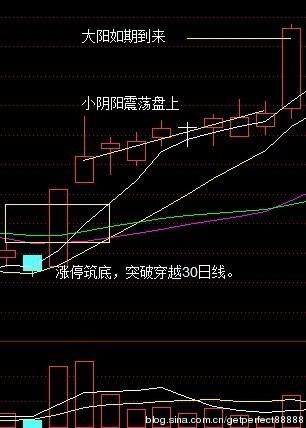B 三泰电子（002312）：2012年2月8日该股涨停，突破30日线，同时穿越30日线，30日线走平（主力建仓，股票由下跌趋势进入筑底趋势），随后该股以小阴阳方式震荡盘上，重心渐次抬高，同时在涨停板实体上方。2月27日，28日，该股出现大涨。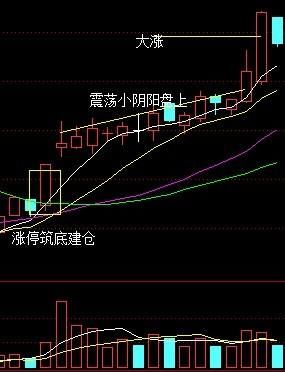C 轴研科技（002064）：2011年6月24日该股涨停，突破30日线，同时穿越30日线，30日线走平（主力建仓，股票由下跌趋势进入筑底趋势），随后该股以小阴阳方式震荡盘上，重心渐次抬高，同时在涨停板实体上方。7月3日，该股出现大涨。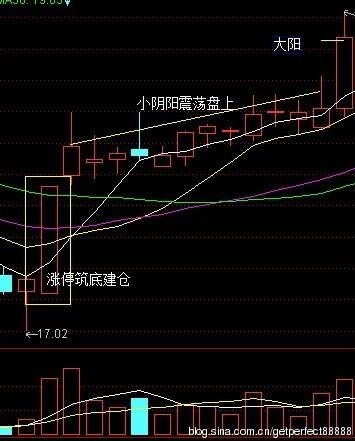1、抓到牛股的概率是0.1%，但是抓到牛回头的概率是80%。

2、抓不到牛股没关系，我们可以抓它牛回头。

3、什么是牛回头？先看一下图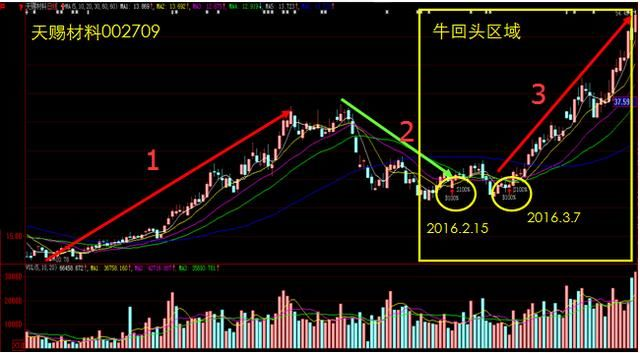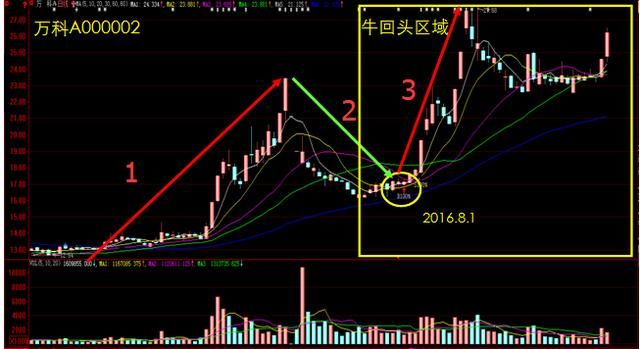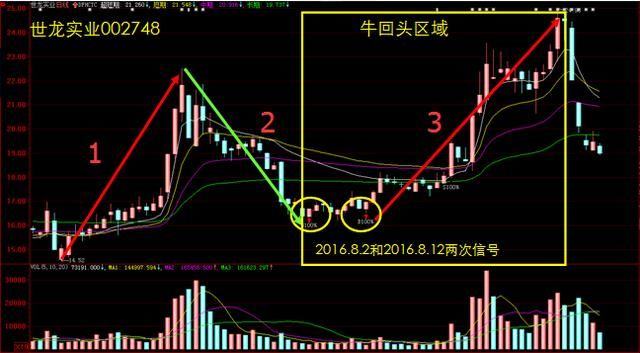A1:=HHV(EMA(C,17),2);

A2:=EMA(C,17),LINETHICK3,COLORRED;

LC:=REF(C,1);

RSV:=(((C-LLV(L,9))/(HHV(H,9)-LLV(L,9)))*100);

K:=SMA(RSV,3,1);

D:=SMA(K,3,1);

J:=((3*K)-(2*D));

RSI:=((SMA(MAX((C-LC),0),9,1)/SMA(ABS((C-LC)),9,1))*100);

DB1:=(RSI>=75);

DB2:=(J>=95);

B1:=(EMA(C,12)-EMA(C,26));

B2:=EMA(B1,3);

ZF:=(((C-REF(C,1))/REF(C,1))*100);

RSI1:=((SMA(MAX((C-LC),0),9,1)/SMA(ABS((C-LC)),9,1))*100);

TDSZ:=(((((((B1>B2) OR (B1=B2)) AND (ZF>9)) AND (ZF

RSV13:=(((C-LLV(L,13))/(HHV(H,13)-LLV(L,13)))*100);

B11:=SMA(RSV13,4,1);

B22:=SMA(B11,3,1);

CDTJ:=(((C>=O) AND CROSS(B11,B22)) AND (B22

TOPB:=IF(((DB1 OR DB2) AND (((C+A2)/2)BOTB:=IF(((CDTJ OR TDSZ) AND (((C+A2)/2)>A2)),(((3*A2)-C)/2),((C+A2)/2));XG:CDTJ,COLORYELLOW;

﻿